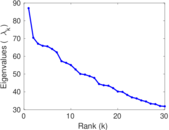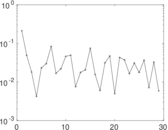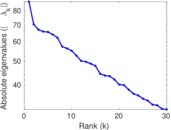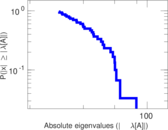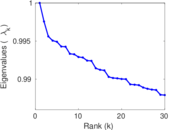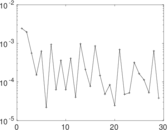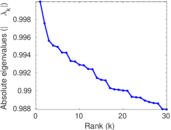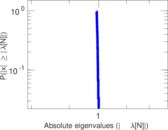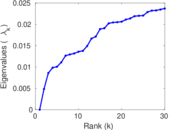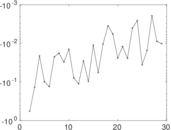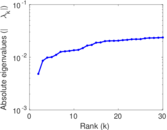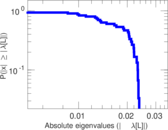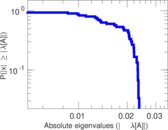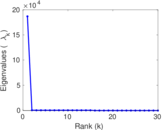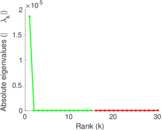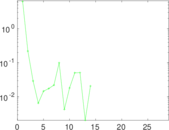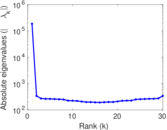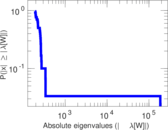# Record labels

This is the bipartite network of musical artists and their record labels from DBpedia. Nodes in the network are artists and record labels. Each edge connects an artist with a record label under which the artist has performed. The edges correspond to the <http://dbpedia.org/ontology/recordLabel> relationships in DBpedia.

 Code `RL` Internal name `dbpedia-recordlabel` Name Record labels Data source http://wiki.dbpedia.org/Downloads AvailabilityDataset is available for download Consistency checkDataset passed all tests Category Affiliation network Dataset timestamp 2001 ⋯ 2017 Node meaning Artist, record Edge meaning Membership Network formatBipartite, undirected Edge typeUnweighted, no multiple edges

## Statistics

 Size n = 186,758 Left size n1 = 168,337 Right size n2 = 18,421 Volume m = 233,286 Wedge count s = 144,874,235 Claw count z = 188,705,350,220 Cross count x = 238,923,687,286,069 Square count q = 1,086,886 4-Tour count T4 = 588,686,820 Maximum degree dmax = 7,446 Maximum left degree d1max = 33 Maximum right degree d2max = 7,446 Average degree d = 2.498 27 Average left degree d1 = 1.385 83 Average right degree d2 = 12.664 1 Fill p = 7.523 08 × 10−5 Size of LCC N = 169,462 Diameter δ = 23 50-Percentile effective diameter δ0.5 = 5.105 38 90-Percentile effective diameter δ0.9 = 6.437 99 Median distance δM = 6 Mean distance δm = 5.326 39 Gini coefficient G = 0.597 056 Balanced inequality ratio P = 0.269 733 Left balanced inequality ratio P1 = 0.418 349 Right balanced inequality ratio P2 = 0.124 551 Relative edge distribution entropy Her = 0.835 910 Power law exponent γ = 4.755 84 Tail power law exponent γt = 2.571 00 Tail power law exponent with p γ3 = 2.571 00 p-value p = 0.000 00 Left tail power law exponent with p γ3,1 = 5.761 00 Left p-value p1 = 0.793 000 Right tail power law exponent with p γ3,2 = 1.711 00 Right p-value p2 = 0.000 00 Degree assortativity ρ = −0.047 893 7 Degree assortativity p-value pρ = 1.607 40 × 10−118 Spectral norm α = 87.074 8 Algebraic connectivity a = 0.004 835 56 Spectral separation |λ1[A] / λ2[A]| = 1.235 64 Controllability C = 153,693 Relative controllability Cr = 0.823 257

## Plots

### Fruchterman–Reingold graph drawing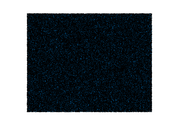### Degree distribution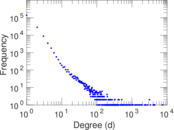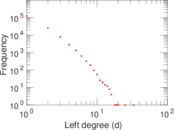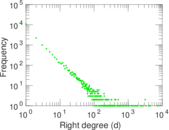### Cumulative degree distribution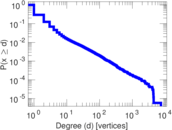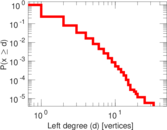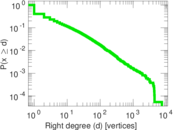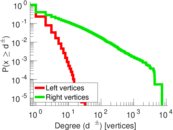### Lorenz curve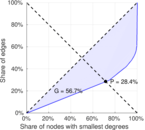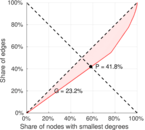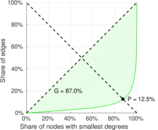### Spectral distribution of the adjacency matrix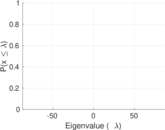### Spectral distribution of the normalized adjacency matrix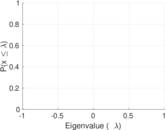### Spectral distribution of the Laplacian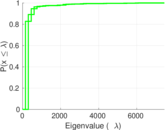### Spectral graph drawing based on the adjacency matrix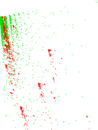### Spectral graph drawing based on the Laplacian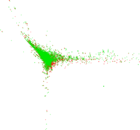### Spectral graph drawing based on the normalized adjacency matrix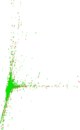### Degree assortativity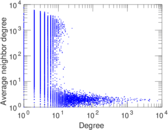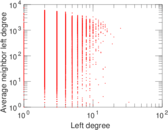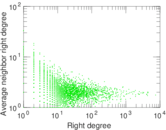### Zipf plot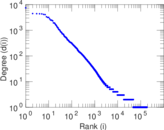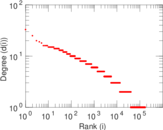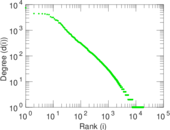### Hop distribution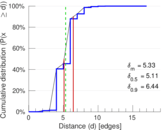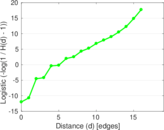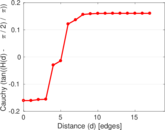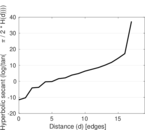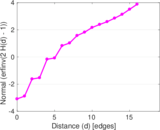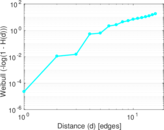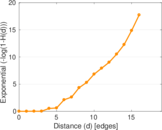### Double Laplacian graph drawing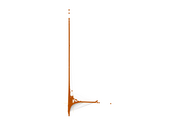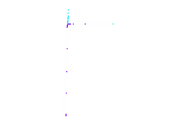### Delaunay graph drawing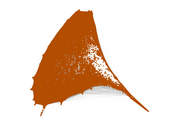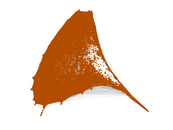### Matrix decompositions plots